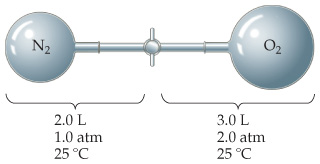# Problem: Consider the apparatus shown in the drawing.What is the total pressure in the container after the gases mix?

###### FREE Expert Solution

Recall that the ideal gas law is:

$\overline{){\mathbf{PV}}{\mathbf{=}}{\mathbf{nRT}}}$

86% (479 ratings)###### Problem Details

Consider the apparatus shown in the drawing.

What is the total pressure in the container after the gases mix?

Frequently Asked Questions

What scientific concept do you need to know in order to solve this problem?

Our tutors have indicated that to solve this problem you will need to apply the Partial Pressure concept. You can view video lessons to learn Partial Pressure. Or if you need more Partial Pressure practice, you can also practice Partial Pressure practice problems.